Signal ProcessingShowing results 31 to 40 of 69, on page 4 of 7 Fourier Transform Pairs Fourier Transform Properties Laplace Transform Pairs Laplace Transform Properties Z Transform Pairs Z Transform Properties
 DESCRIPTION EQUATION Laplace transform involving the unit step function, cosine, and an exponential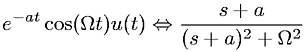Laplace transform involving the unit step function, sine, and an exponential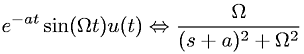Laplace transform linearity property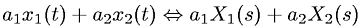Laplace transform Nth time domain derivative propertyLaplace transform of Kroeneker delta functionLaplace transform of unit step function times a constant (K)Laplace transform time domain linear convolution (s domain multiplication) property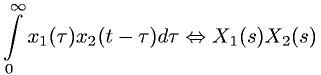Laplace transform time domain scaling property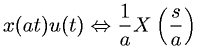Laplace transform time domain shifting property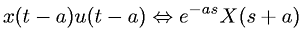Laplace transform time varying coefficient (s domain differentiation) propertyGo to page: 1  2  3  4  5  6  7